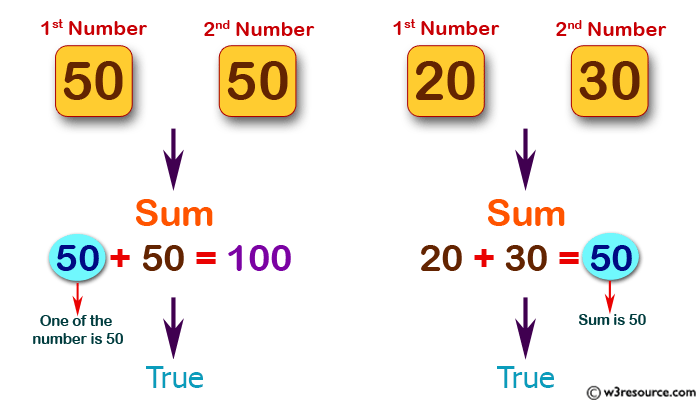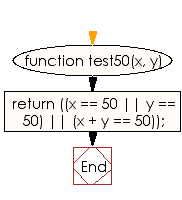# JavaScript: Check two given numbers and return true if one of the number is 50 or if their sum is 50

## JavaScript Basic: Exercise-18 with Solution

Write a JavaScript program to check two given numbers and return true if one of the number is 50 or if their sum is 50.

Pictorial Presentation:Sample Solution:

HTML Code:

``````<!DOCTYPE html>
<html>
<meta charset="utf-8">
<meta name="viewport" content="width=device-width">
<title>JavaScript program to check two given numbers and return true if one of the number is 50 or if their sum is 50.</title>
<body>

</body>
</html>
```
```

JavaScript Code:

``````function test50(x, y)
{
return ((x == 50 || y == 50) || (x + y == 50));
}
console.log(test50(50, 50))
console.log(test50(20, 50))
console.log(test50(20, 20))
console.log(test50(20, 30))
```
```

Sample Output:

```true
true
false
true
```

Flowchart:ES6 Version:

``````function test50(x, y)
{
return ((x == 50 || y == 50) || (x + y == 50));
}
console.log(test50(50, 50))
console.log(test50(20, 50))
console.log(test50(20, 20))
console.log(test50(20, 30))
``````

Live Demo:

See the Pen JavaScript: Check two given numbers- basic-ex-18 by w3resource (@w3resource) on CodePen.

Improve this sample solution and post your code through Disqus

What is the difficulty level of this exercise?

Test your Programming skills with w3resource's quiz.

﻿

## JavaScript: Tips of the Day

Returns the sum of the powers of all the numbers from start to end (both inclusive)

Example:

```const sumPower = (end, power = 2, start = 1) =>
Array(end + 1 - start)
.fill(0)
.map((x, i) => (i + start) ** power)
.reduce((a, b) => a + b, 0);
console.log(sumPower(10)); // 385
console.log(sumPower(10, 3)); // 3025
console.log(sumPower(10, 3, 5)); // 2925
```

Output:

```385
3025
2925
```